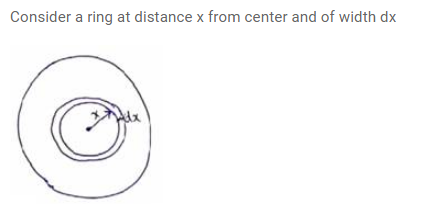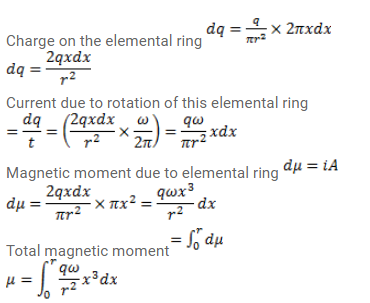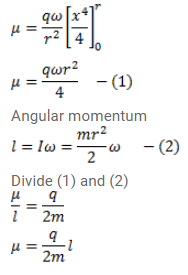# Consider a nonconducting plate of radius r and

Question:

Consider a nonconducting plate of radius $r$ and mass $m$ which has a charge $q$ distributed uniformly over it. The plate is rotated about its axis with an angular speed $\omega$. Show that the magnetic moment $\mu$ and the angular momentum $\mid$ of the plate are related as $\mu=\frac{q}{2 m} l$.

Solution: